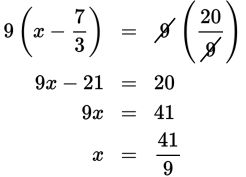# SAT Math Multiple Choice Question 293: Answer and Explanation

### Test Information

Question: 293

8. Which value of x makes the equation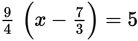true?

• A.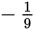• B.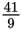• C.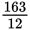• D.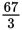Explanation:

B

Difficulty: Medium

Category: Heart of Algebra / Linear Equations

Strategic Advice: This question has multiple fractions, so start by clearing the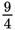by multiplying both sides of the equation by its reciprocal,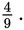Getting to the Answer: You might have to repeat this process to eliminate any remaining fractions.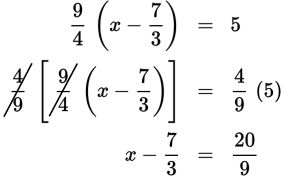There are still a couple of fractions in the equation, so multiply by the common denominator this time, which is 9.